# Ω 3

Value LearningAI
Frontpage
Crossposted from the AI Alignment Forum. May contain more technical jargon than usual.

This post was written for Convergence Analysis by Michael Aird, based on ideas from Justin Shovelain and with ongoing guidance from him. Throughout the post, “I” will refer to Michael, while “we” will refer to Michael and Justin or to Convergence as an organisation.

Epistemic status: High confidence in the core ideas on an abstract level. Claims about the usefulness of those ideas, their practical implications, and how best to concretely/mathematically implement them are more speculative; one goal in writing this post is to receive feedback on those things. I’m quite new to many of the concepts covered in this post, but Justin is more familiar with them.

# Overview

This post outlines:

• What vector fields are
• How they can be used to visualise preferences
• How utility functions can be generated from “preference vector fields” (PVFs)
• How PVFs can be extrapolated from limited data on preferences
• How to visualise inconsistent preferences (as “curl”)
• A rough idea for how to “remove curl” to generate consistent utility functions
• Possible areas for future research

We expect this to provide useful tools and insights for various purposes, most notably AI alignment, existential risk strategy, and rationality.

This post is structured modularly; different sections may be of interest to different readers, and should be useful in isolation from the rest of the post. The post also includes links to articles and videos introducing relevant concepts, to make the post accessible to readers without relevant technical backgrounds.

# Vector fields and preferences

A vector represents both magnitude and direction; for example, velocity is a vector that represents not just the speed at which one is travelling but also the direction of travel. A vector field essentially associates a vector to each point in a region of space. For example, the following image (source) shows the strength (represented by arrow lengths) and direction of the magnetic field at various points around a bar magnet: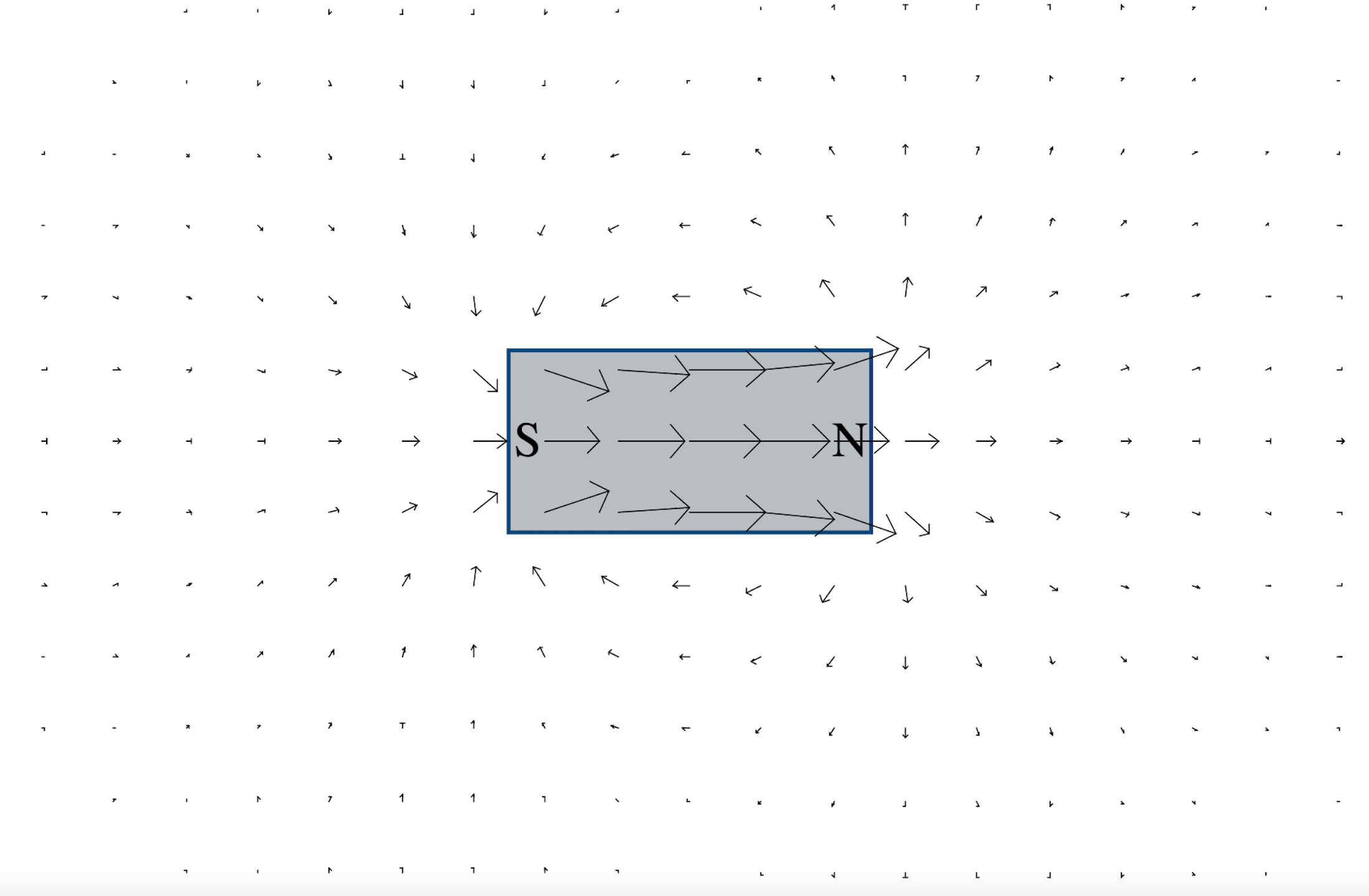Figure 1.

Another common usage of vector fields is to represent the direction in which fluid would flow, for example the downhill flow of water on uneven terrain (this short video shows and discusses that visualisation).

We believe that vector fields over “state spaces” (possible states of the world, represented by positions along each dimension) can be a useful tool for analysis and communication of various issues (e.g., existential risk strategy, AI alignment). In particular, we’re interested in the idea of representing preferences as “preference vector fields” (PVFs), in which, at each point in the state space, a vector represents which direction in the state space an agent would prefer to move from there, and how intense that preference is. (For the purposes of this post, “agent” could mean an AI, a human, a community, humanity as a whole, etc.)

To illustrate this, the following PVF shows a hypothetical agent’s preferences over a state space in which the only dimensions of interest are wealth and security.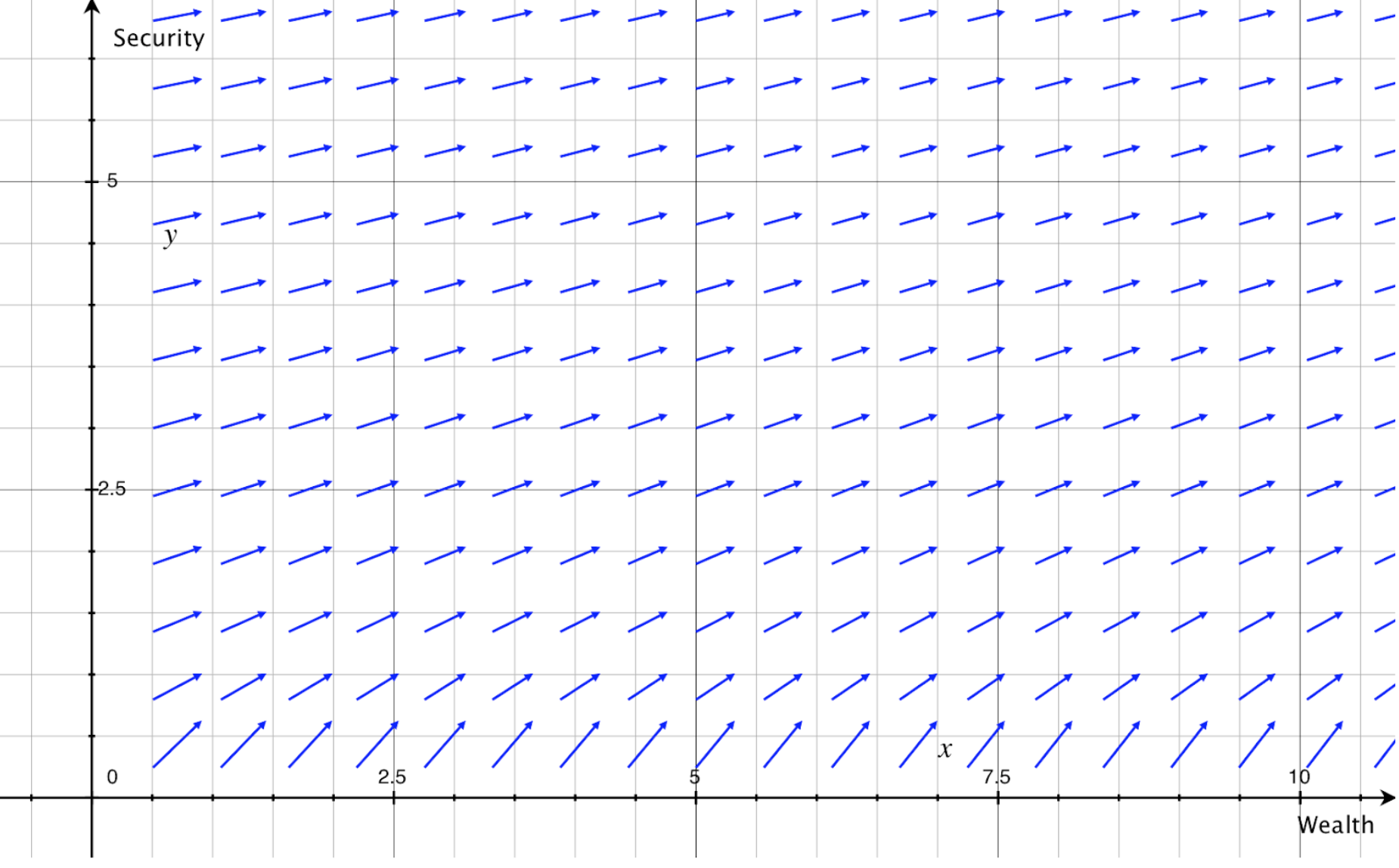Figure 2.

The fact that (at least over the domain shown here) the arrows always point at least slightly upwards and to the right shows that the agent prefers more wealth and security to less, regardless of the current level of those variables. The fact that the arrows are longest near the x axis shows that preferences are most intense when security is low. The fact that the arrows become gradually more horizontal as we move up the y axis shows that, as security increases, the agent comes to care more about wealth relative to security.

## Not only preferences

In a very similar way, vector fields can be used to represent things other than preferences. For example, we might suspect that for many agents (e.g., most/all humans), preferences do not perfectly match what would actually make the agent happier (e.g., because of the agent being mistaken about something, or having separate systems for reward vs motivation). In this case, we could create a vector field to represent the agent’s preferences (represented by the blue arrows below), and another to represent what changes from any given point would increase the agent’s happiness (represented by the green arrows).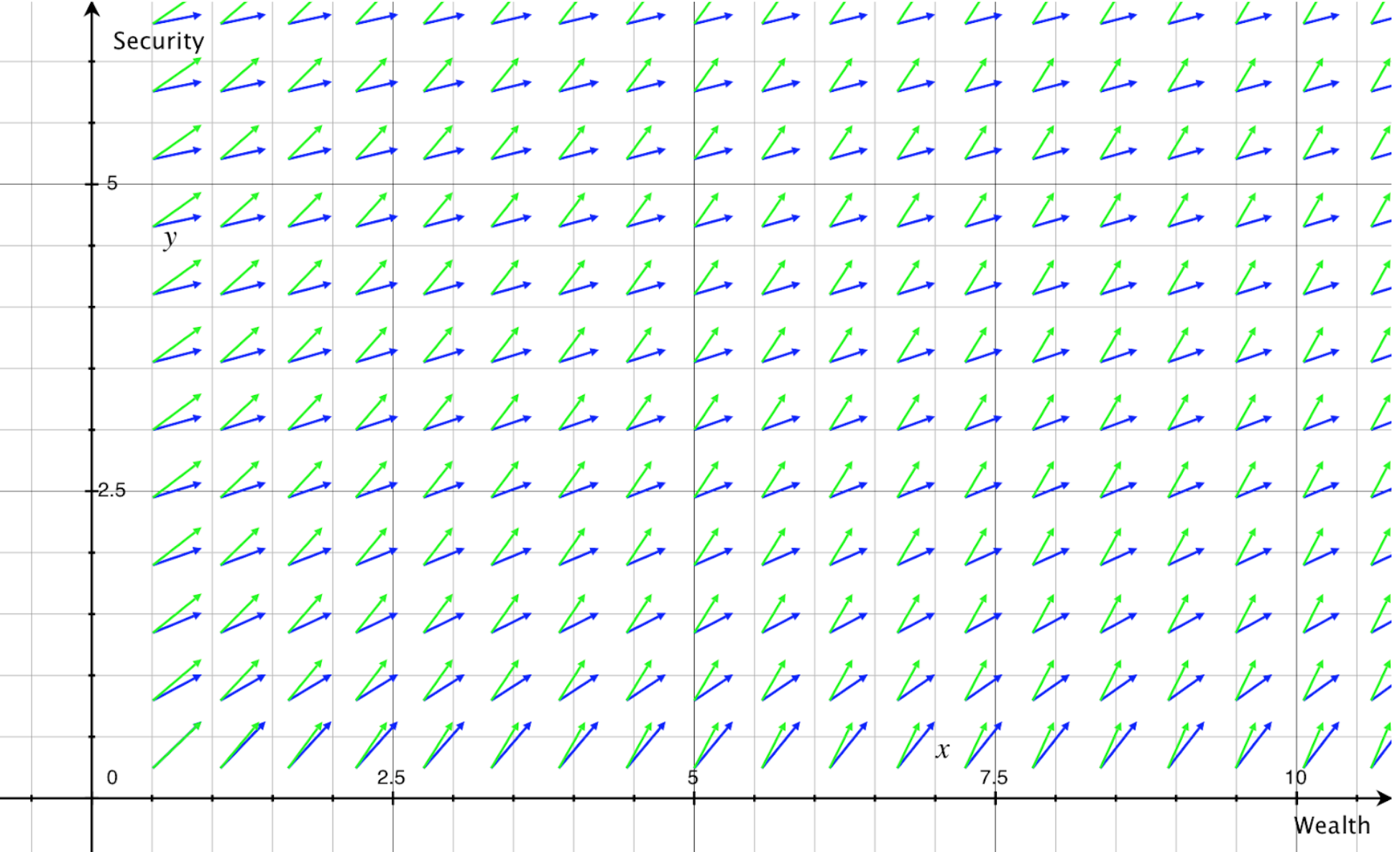Figure 3.

This method of layering vector fields representing different things can be used as one tool in analysing potential clashes between different things (e.g., between an agent’s preferences and what would actually make the agent happy, or between an agent’s beliefs about what changes would be likely at each state and what changes would actually be likely at each state).

For example, the above graph indicates that, as wealth and/or security increases (i.e., as we move across the x axis and/or up the y axis), there is an increasing gap between the agent’s preferences and what would make the agent happy. In particular, security becomes increasingly more important than wealth for the agent’s happiness, but this is not reflected in the agent’s preferences.

(Note that, while it does make sense to compare the direction in which arrows from two different vector fields point, I haven’t yet thought much about whether it makes sense to compare the lengths Grapher shows for their arrows. It seems like this is mathematically the same as the common problem of trying to compare utility functions across different agents, or preferences across different voters. But here the functions represent different things within the same agent, which may make a difference.)

When a vector field has no “curl” (see the section “Curl and inconsistent preferences” below), the vector field can be thought of as the gradient of a scalar field. (A scalar field is similar to a vector field, except that it associates a scalar with each point in a region of space, and scalars have only magnitude, rather than magnitude and direction.) Essentially, this means that the arrows of the vector field can be thought of as pointing “uphill”, away from low points and towards high points of the associated scalar function. If the vector field represents preferences, higher points of the scalar function would be where preferences are more satisfied, and lower points are where it is less satisfied; thus, the scalar function can be thought of as the agent’s utility function. (The same basic method is often used in physics, in which context the scalar function typically represents scalar potential.)

Below is one visualisation of the scalar field representing the utility function of the agent from the previous example (based on its preferences, not on what would make it “happy”), as well as the related vector field. Colours towards the red end of the spectrum represent higher values of the scalar field. It can be seen that the arrows of the vector field point away from blue areas and towards red areas, representing the agent’s preference for “climbing uphill” on its utility function.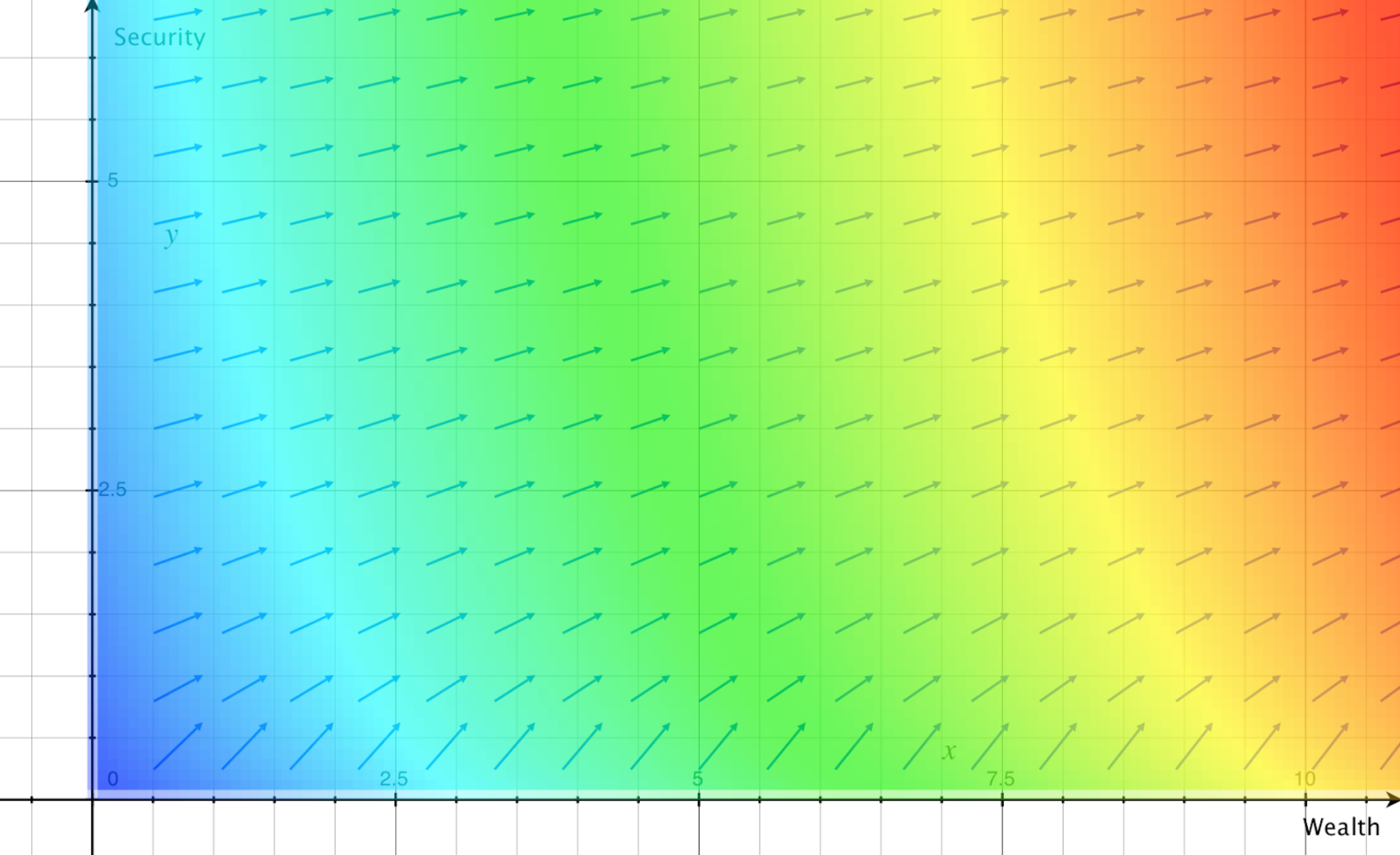Figure 4.

The scalar field can also be represented in three dimensions, as values on the z dimension, which are in turn a function of values on the x and y dimensions. This is shown below (from two angles), for the same agent. (These graphs are a little hard to interpret from still images on a 2D screen, at least with this function; such graphs can be easier to interpret when one is able to rotate the angle of view.)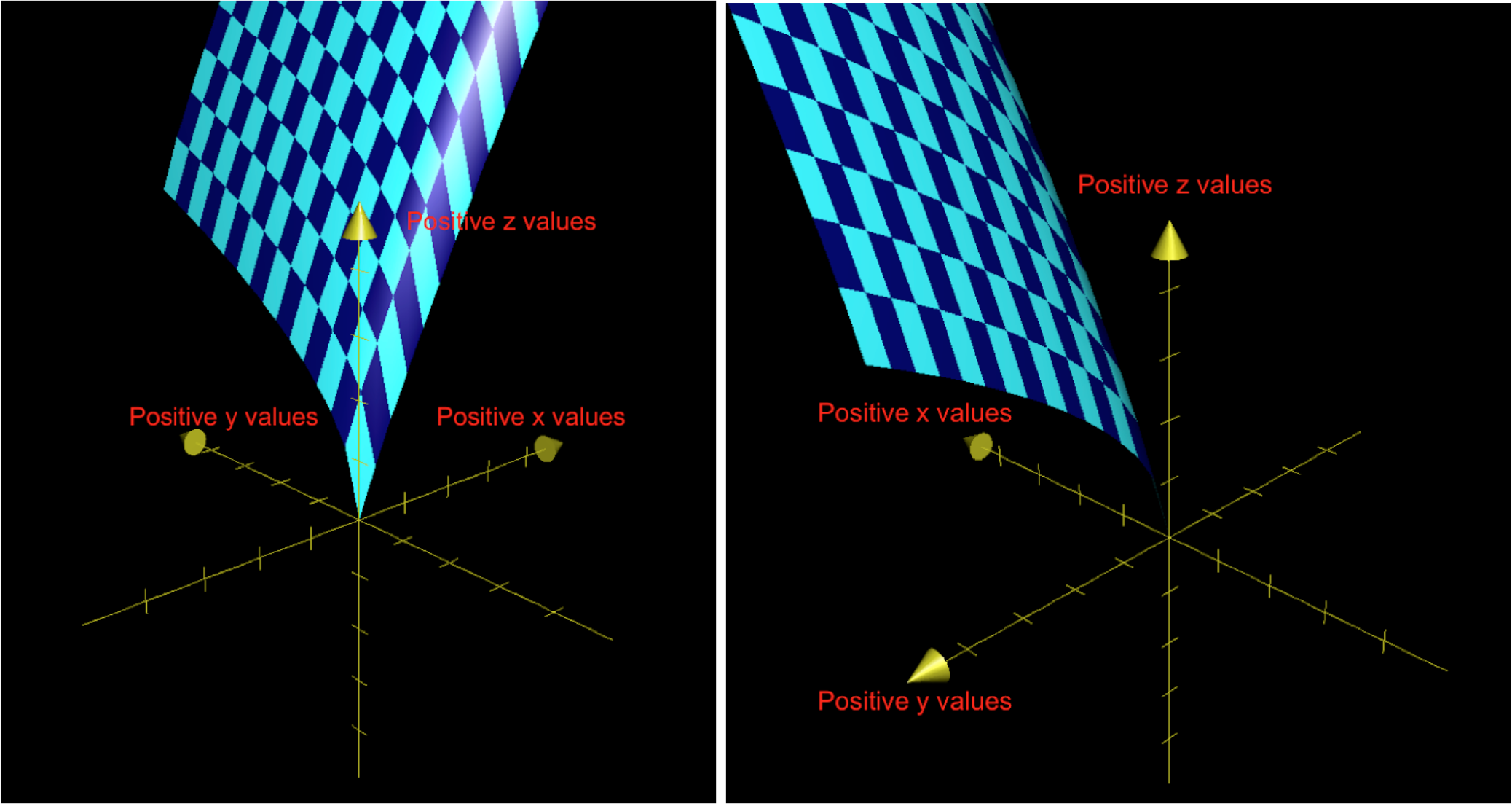Figures 5a and 5b.

## Method

This video provides one clear explanation of the actual method for determining the scalar function that a curl-free vector field can be thought of as the gradient of (though the video is focused on cases of 3D vector fields). That video describes this as finding the “potential”; as noted earlier, when the vector field represents preferences, the utility function can be thought of as analogous to the “potential” in other cases.

Personally, as a quick method of finding the scalar function associated with a 2D vector field, I used the following algorithm, from the first answer on this page:

DSolve[{D[f[x, y], x] == [X COMPONENT OF THE VECTOR FIELD], D[f[x, y], y] == [Y COMPONENT OF THE VECTOR FIELD]}, f[x, y], {x, y}]

I input the algorithm into a Wolfram Cloud notebook, which seems to be free to use as long as you create an account. (As noted in the answer on the linked page, this algorithm will come back with no solution if the vector field has curl. This makes sense, because this general approach cannot be used in this way if a field has curl; this is explained in the section “Curl and inconsistent preferences” below.) Finally, I double-checked that the function was a valid solution by using this calculator to find its gradient, which should then be the same as the original vector field.

# Extrapolating PVFs (and utility functions) from specific preference data

In reality, one rarely knows an agent’s actual utility function or their full PVF. Instead, one is likely to only have data on the agent’s (apparent) preferences at particular points in state space; for example, the extent to which they wanted more wealth and more security when they had \$10,000 of savings and a “4/5” level of security.

One can imagine extrapolating a full preference vector field (PVF) from that data. We do not know of a precise method for actually doing this (we plan to do more research and thought regarding that in future). However, conceptually speaking, it seems the process would be analogous to fitting a regression line to observed data points, and, like that process, would require striking a balance between maximising fit with the data and avoiding overfitting.

For an example (based very loosely on Figure 3 in this article), suppose that I know that Alice prefers car A to Car B, Car B to Car C, Car C to Car D, and Car D to Car A (i.e., to Alice, A>B>C>D>A). I also know the weight (in thousands of pounds) and perceived “sportiness” (as rated by consumers) of the four cars, and am willing to make the simplifying assumption that these are the only factors that influenced Alice’s preferences. I could then create a plane with weight on the x axis and sportiness on the y axis, show the position of the four cars in this space, and represent Alice’s preferences with arrows pointing from each car towards the car Alice would prefer to that one, as shown below: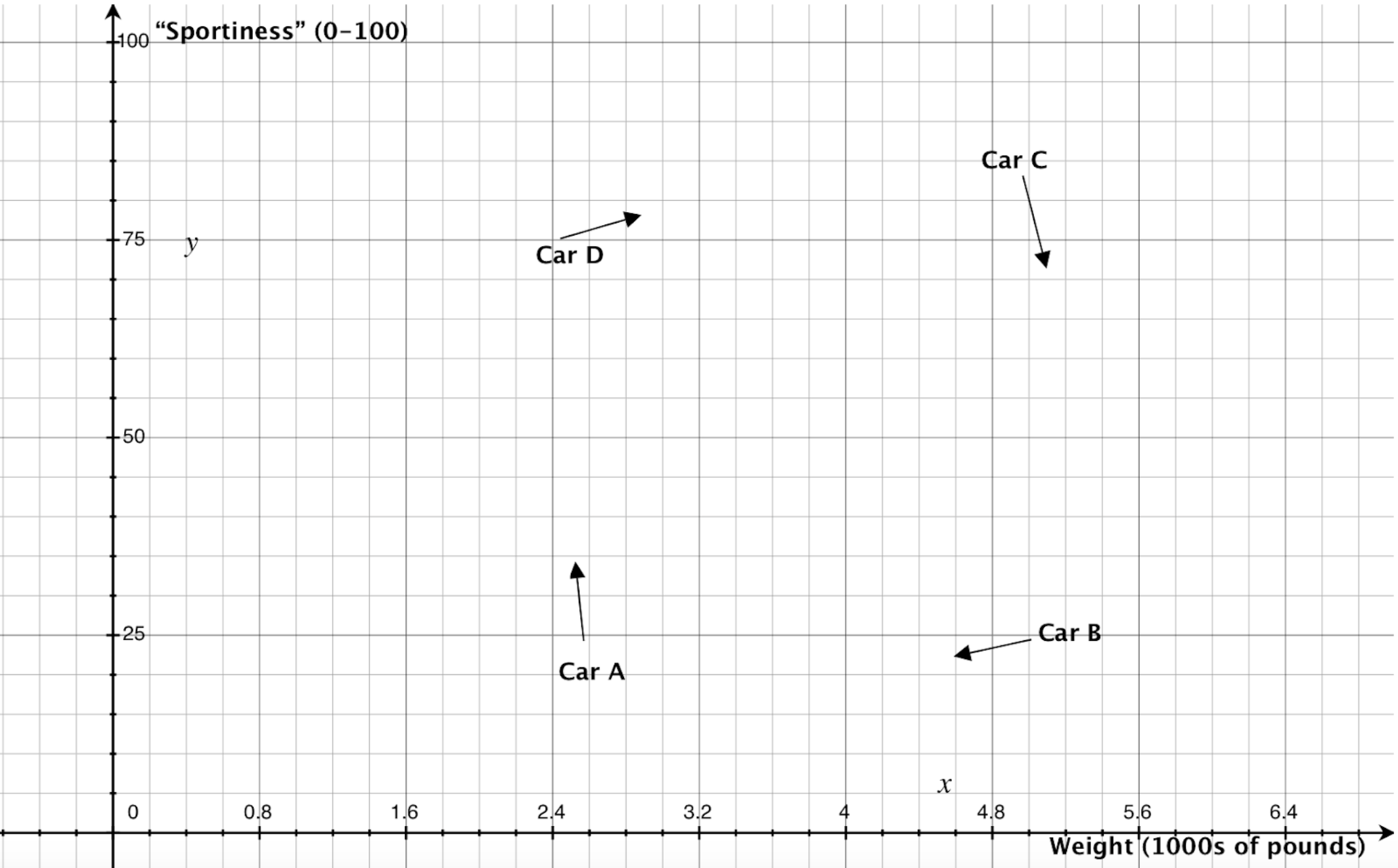Figure 6.

I could then infer a PVF that (1) approximately captures Alice’s known preferences, and (2) suggests what preferences Alice would have at any other point in the plane (rather than just at the four points I have data for). In this case, one seemingly plausible PVF is shown below, with the length of each blue arrow representing the strength of Alice’s preferences at the associated point. (This PVF still shows Alice’s known preferences, but this is just for ease of comparison; those known preferences are not actually part of the PVF itself.)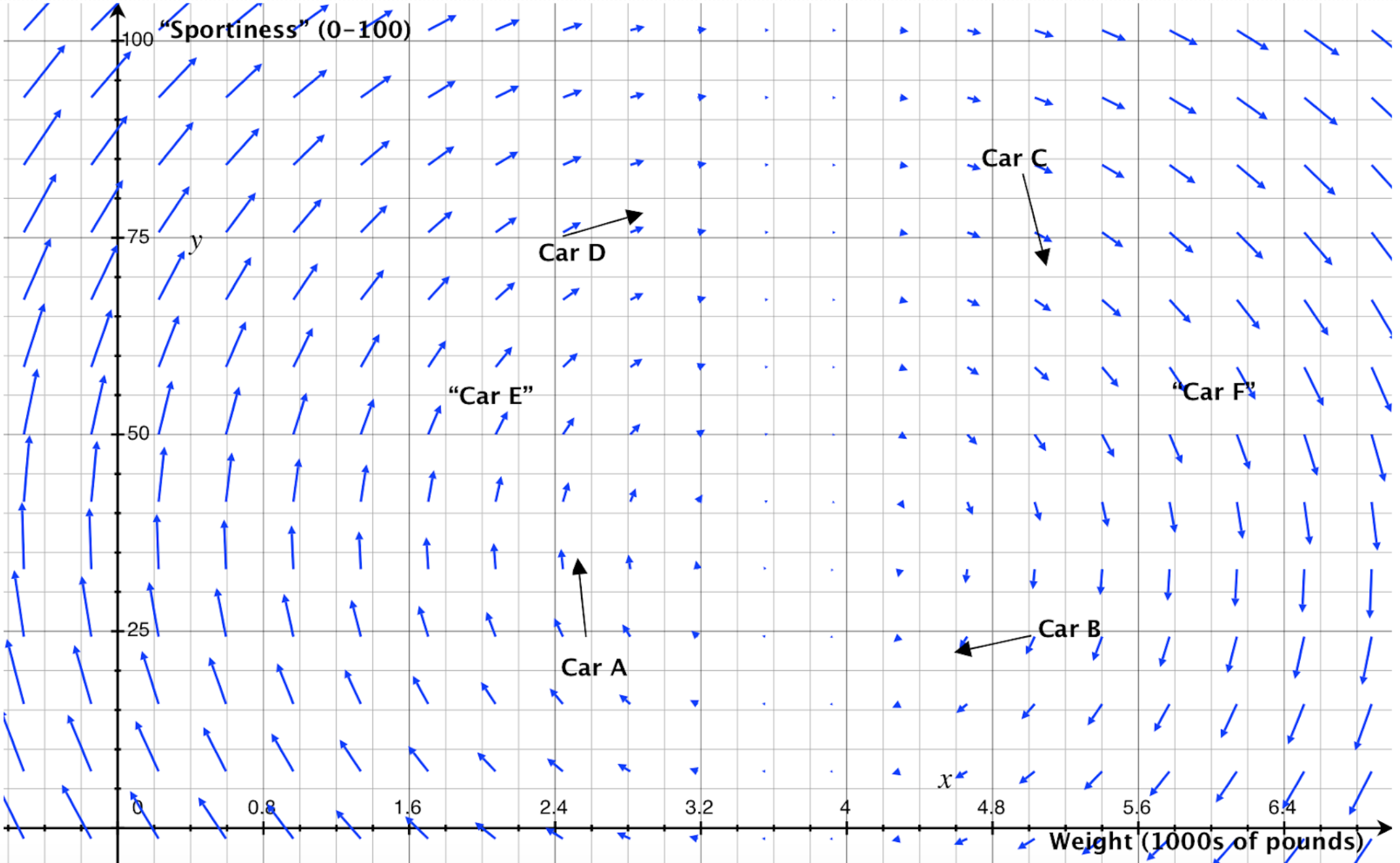Figure 7.

This PVF allows us to make predictions about what Alice’s preferences would be even in situations we do not have any empirical data about. For example, this PVF suggests that if Alice had the hypothetical car E (with a weight of ~2000 pounds and sportiness of ~55), she would prefer a car that was heavier and was higher for sportiness. In contrast, the PVF also suggests that, if she had the hypothetical car F (with a weight of ~6000 pounds and sportiness of ~55), she would prefer a car that was heavier and was rated lower for sportiness.

Of course, these predictions are not necessarily accurate. One could likely create many other PVFs that also “appear” to roughly fit Alice’s known preferences, and these could lead to different predictions. This highlights why we wish to find a more precise/“rigorous” method to better accomplish the goal I have conceptually gestured at here.

It’s also worth noting that one could extrapolate an agent’s utility function from limited preference data by first using the method gestured at here and then using the method covered in the previous section. That is, one could gather some data on an agent’s (apparent) preferences, extrapolate a PVF that “fits” that data, and then calculate what (set of) scalar function(s) that vector field is the gradient of. That scalar function would be the agent’s extrapolated utility function.

However, as noted earlier, this method only works if the PVF has no “curl”, so it would not work in the case of Alice’s preferences about cars. I will now discuss what I mean by “curl”, what implications curl has, and a rough idea for “removing” it.

# Curl and inconsistent preferences

In the example above, to Alice, A>B>C>D>A. This is a case of intransitivity, or, less formally, circular or inconsistent preferences. This is typically seen as irrational, and as opening agents up to issues such as being “money pumped”. It seems that Alice would be willing to just keep paying us to let her trade in one car for the one she preferred to that one, and do this endlessly - going around and around in a circle, yet feeling that her preferences are being continually satisfied.

So another pair of reasons why representing preferences as vector fields is helpful is that doing so allows inconsistencies in preferences:

1. to be directly seen (if they are sufficiently extreme)
2. to be calculated as the vector field’s curl

This video introduces the concept of curl. Returning to the visualisation of vector fields as representing the direction in which water would flow over a certain domain, curl represents the speed and direction an object would spin if placed in the water. For example, if there is a strong clockwise curl at a certain point, a stick placed there would rotate clockwise; if there is no curl at a point, a stick placed there would not rotate (though it still may move in some direction, as represented by the vector field itself).

Note that the concepts of curl and inconsistency will also apply in less extreme cases (i.e., where an agent’s preferences do not only “chase each other around in circles”).

As noted earlier, when a vector field has curl, one cannot find its gradient. In our context, this seems logical; if an agent’s preferences are inconsistent, it seems that the agent cannot have a true utility function, and that we can’t assign any meaningful “height” to any point in the 2D state space. Consider again the example of Alice’s preferences for cars; if we were to interpret meeting her preferences as moving “uphill” on a utility function, she could keep arriving back at the same points in the state space and yet be at different “heights”, which doesn’t seem to make sense.

## Removing curl to create consistent utility functions

It seems that agents frequently have intransitive preferences, and thus that their PVFs will often have some curl. It would therefore be very useful to have a method for “removing curl” from a PVF, to translate an intransitive set of preferences into a transitive set of preferences, while making a minimum of changes. This new, consistent PVF would also then allow for the generation of a corresponding utility function for the agent.

We believe that this process should be possible. We also believe that, if developed and confirmed to make sense, it could be useful for various aspects of AI alignment (among other things). In particular, it could help in:

• extrapolation of a consistent “core” (and corresponding utility function) from inconsistent human preferences (which could then inform an AI’s decisions)
• adjustment of an AI’s inconsistent preferences (either by engineers or by the AI itself), with a minimum of changes being made

We have not yet implemented this process for removing curl. But we believe that the Helmholtz theorem should work, at least for PVFs in 3 or fewer dimensions (and we believe that a higher dimensional generalization probably exists). The Helmholtz theorem:

states that any sufficiently smooth, rapidly decaying vector field in three dimensions can be resolved into the sum of an irrotational (curl-free) vector field and a solenoidal (divergence-free) vector field; this is known as the Helmholtz decomposition or Helmholtz representation. (Wikipedia)

This irrotational (curl-free) vector field would then be the consistent projection (in a CEV-like way) of the agent’s preferences (from which the agent’s utility function could also be generated, in the manner discussed earlier).

# Uncertainties and areas for further research

The following are some areas we are particularly interested in getting comments/feedback on, seeing others explore, or exploring ourselves in future work:

• Are there any flaws or misleading elements in the above analysis? (As noted earlier, this is essentially just an initial exploration of some tools/concepts.)
• To what extent do the methods used and claims made in this post generalise to higher-dimensional spaces (e.g., when we wish to represent preferences over more than two factors at the same time)? To what extent do they generalise to graphs of states that don’t correspond to any normal geometry?
• Is there an existing, rigorous/precise method for extrapolating a PVF from a limited number of known preferences (or more generally, extrapolating a vector field from a limited number of known vectors)? If not, can a satisfactorily rigorous/precise method be developed?
• Are there meaningful and relevant differences between the concepts of curl in vector fields and of intransitivity, inconsistency, irrationality, and incoherence in preferences? If so, how does that change the above analysis?
• Is it possible to “remove curl” in the way we want, in the sort of situations we’re interested in (in particular, not only in three dimensions)? If so, how, specifically?
• What other implications do the above ideas have? E.g., for rationality more generally, or for how to interpret and implement preference utilitarianism. (Above, I mostly just introduced the ideas, and hinted at a handful of implications.)
• What other uses could these “tools” be put to?

1. It appears some prior work (e.g., this and this) has explored the use of vector fields to represent preferences. Unfortunately, I haven’t yet had time to investigate this work, so there may be many useful insights in there that are lacking in this post. ↩︎

2. Of course, there are often far more than two key factors influencing our preferences. In such cases, a vector field over more dimensions can be used instead (see here for an introduction to 3D vector fields). I focus in this post on 2D vector fields, simply because those are easier to discuss and visualise. We expect many of the ideas and implications covered in this post will be similar in higher dimensional vector fields, but we aren’t yet certain about that, and intend to more carefully consider it later. ↩︎

3. For both this example and most others shown, the precise equations used were chosen quite arbitrarily, basically by trying equations semi-randomly until I found one that roughly matched the sort of shape I wanted. For those interested, I have screenshots of all equations used, in their order of appearance in this post, here. To create the visuals in this post, I entered these equations into Grapher (for those interested in trying to do similar things themselves, I found this guide useful). I discuss below, in the section “Extrapolating PVFs (and utility functions) from specific preference data”, the issue of how to actually generate realistic/accurate PVFs in the first place. ↩︎

4. It’s possible that here I’m conflating the concepts of conservative, irrotational, and curl-free vector fields in a way that doesn’t make sense. If any readers believe this is the case, and especially if they believe this issue changes the core ideas and implications raised in this post, I would appreciate them commenting or messaging me. ↩︎

5. Technically, the vector field is the gradient of a class of functions, with the functions differing only in their constant term. This is because gradient only relates to differences in height (or roughly analogous ideas, in higher-dimensional cases), not to absolute heights. One can imagine raising or lowering the entire scalar function by the same constant without affecting the gradient between points. (I show in this document examples of what this would look like, while in this post itself I keep all constants at 0.) Thus, in one sense, a PVF does not fully specify the associated utility function representation, but the constant can be ignored anyway (as utility functions are unique up to positive affine transformations). ↩︎

6. I have purposefully chosen a set of circular (or “intransitive”) preferences, as the next session will use this example in discussing the problem of circularity and how to deal with it. ↩︎

7. Note that, in this example, I am not assuming any knowledge about the strength of Alice’s preferences, only about their direction. As such, the length of the arrows representing Alice’s known preferences has no particular meaning. ↩︎

8. In conversation with Justin, Linda Linsefors mentioned having had a somewhat similar idea independently. ↩︎